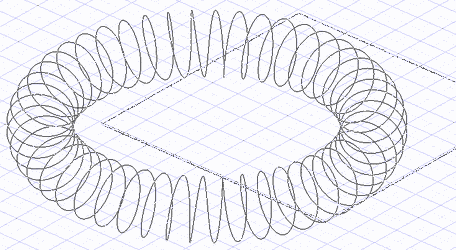# Curve from Law

(Available only in Platinum)

Default UI Menu: Draw/3D Object/3D Law Objects/Curve from Law Ribbon UI Menu: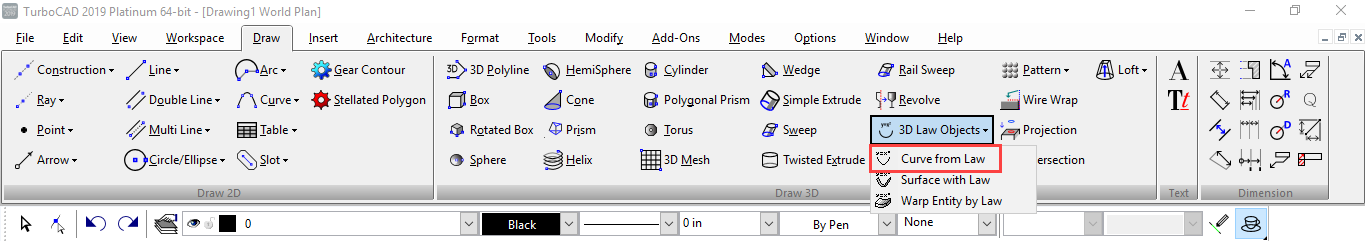This tool creates an 2D,3D Curve using Laws. The resulting curve can be used by the Graphic on Path tool and in Sweeps etc. Laws are mathematical functions in the form of a formula. They are used to define how resulting objects are modified. They are composed and selected in the Laws Editor which is specific to each tool that uses laws and can be opened from that tool.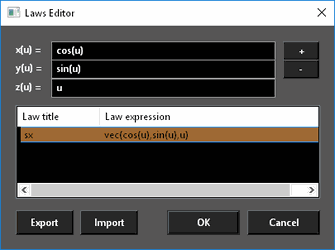To use a the Curve from Law tool:

1. Select the tool. The Laws Editor opens.
2. Select the desired law in the Laws table by double-clicking and press OK. The law that is applied is what is in the formula field.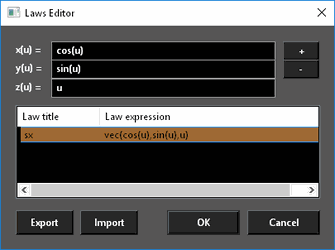3. Set the U-min and U-max either by entering a values in the Inspector Bar or by selecting two points. (U-min defaults to zero (0))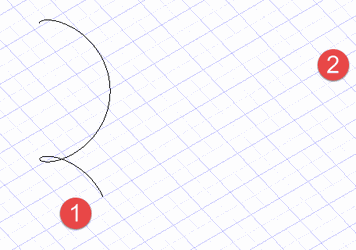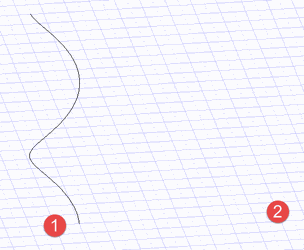To create a law:

1. Type the desired formulas into the formula fields.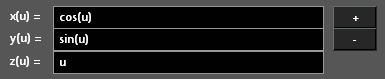1. Press the + (plus) button.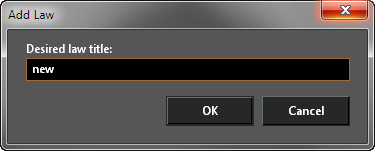1. Type in a titke for the law and press OK. The new law will appear in the Laws table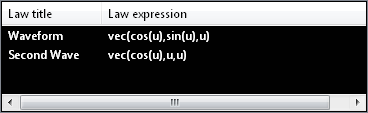To Delete a law:

1. Open the Laws Editor.

2. Click on the Law in the Laws table to select it.

3. Right click and select Delete, or press the - (minus) button.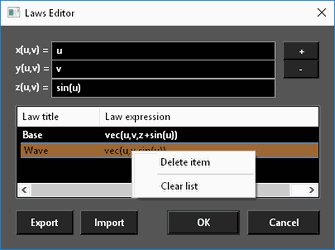Laws cannot be edited but double click a law will load it into the Formula fields where it can be altered and then a new altered law added.

To Delete all laws:

1. Open the Laws Editor.

2. Click on the Law in the Laws table to select it.

3. Right click and select Clear List.To Export laws:

1. Open the Laws Editor.
2. Click Export.
3. Type a file name and click Save.To Import laws:

1. Open the Laws Editor.
2. Click Import.
3. Select a file and click Open.Laws cannot be edited but double click a law will load it into the Formula fields where it can be altered and then a new altered law added.

Editing a Curve by Law

The values for a Curve by law can be edited via the Selection Info palette or by the object properties.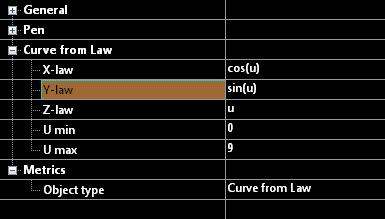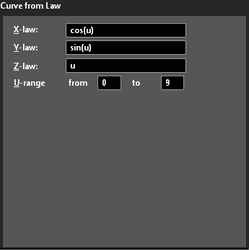x(u) y(u) z(u)

In the Offset tool the function are defined as a function of U. Where U is the distance along the object.

Examples:

Setting x(u)=cos(u), y(u)=u, z(u)=u, U min = 0, U max = 9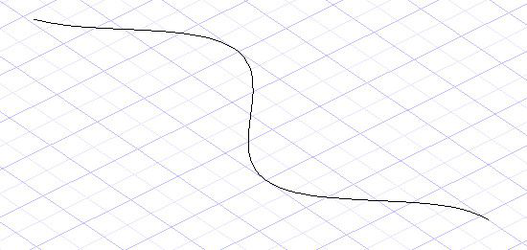Setting x(u)=cos(u), y(u)=sin(u), z(u)=u, U min = 0, U max = 9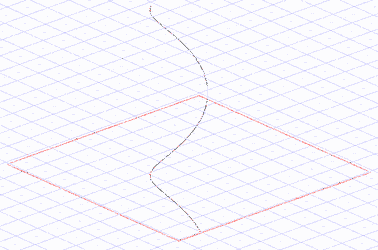Setting x(u)=(5+cos(u))cos(u/48), y(u)=(5+cos(u))sin(u/48), z(u)=sin(u), U min = 0, U max = 301.579987